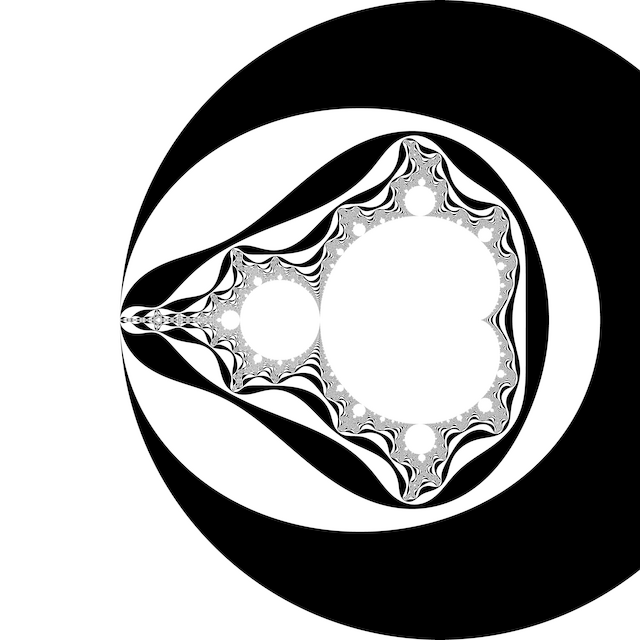# # Escape TimeColour according to the integer number of iterations $$n$$ at which $\left|z_n\right| > R \ge \left|z_{n-1}\right|$ where escape radius $$R \ge 2$$.

## # 1 C99 Code

#include <complex.h>

int m_escape_time(int N, double R, double _Complex c)
{
double _Complex z = 0;
for (int n = 0; n < N; ++n)
{
if (cabs(z) > R)
return n;
z = z * z + c;
}
return -1;
}